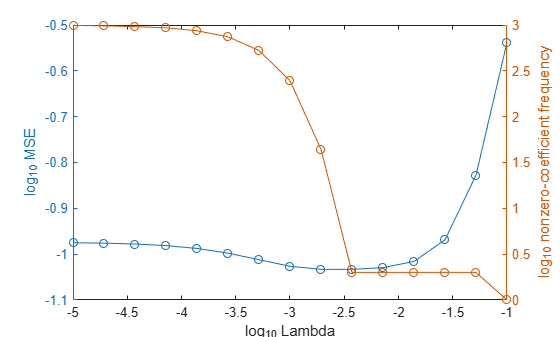# predict

Class: RegressionLinear

Predict response of linear regression model

## Syntax

``YHat = predict(Mdl,X)``
``YHat = predict(Mdl,X,Name,Value)``

## Description

example

````YHat = predict(Mdl,X)` returns predicted responses for each observation in the predictor data `X` based on the trained linear regression model `Mdl`. `YHat` contains responses for each regularization strength in `Mdl`.```

example

````YHat = predict(Mdl,X,Name,Value)` returns predicted responses with additional options specified by one or more `Name,Value` pair arguments. For example, specify that columns in the predictor data correspond to observations.```

## Input Arguments

expand all

Linear regression model, specified as a `RegressionLinear` model object. You can create a `RegressionLinear` model object using `fitrlinear`.

Predictor data, specified as an n-by-p full or sparse matrix. This orientation of `X` indicates that rows correspond to individual observations, and columns correspond to individual predictor variables.

### Note

If you orient your predictor matrix so that observations correspond to columns and specify `'ObservationsIn','columns'`, then you might experience a significant reduction in computation time.

The length of `Y` and the number of observations in `X` must be equal.

Data Types: `single` | `double`

### Name-Value Pair Arguments

Specify optional comma-separated pairs of `Name,Value` arguments. `Name` is the argument name and `Value` is the corresponding value. `Name` must appear inside quotes. You can specify several name and value pair arguments in any order as `Name1,Value1,...,NameN,ValueN`.

Predictor data observation dimension, specified as the comma-separated pair consisting of `'ObservationsIn'` and `'columns'` or `'rows'`.

### Note

If you orient your predictor matrix so that observations correspond to columns and specify `'ObservationsIn','columns'`, then you might experience a significant reduction in optimization-execution time.

## Output Arguments

expand all

Predicted responses, returned as a n-by-L numeric matrix. n is the number of observations in `X` and L is the number of regularization strengths in `Mdl.Lambda`. `YHat(i,j)` is the response for observation `i` using the linear regression model that has regularization strength `Mdl.Lambda(j)`.

The predicted response using the model with regularization strength j is ${\stackrel{^}{y}}_{j}=x{\beta }_{j}+{b}_{j}.$

• x is an observation from the predictor data matrix `X`, and is row vector.

• ${\beta }_{j}$ is the estimated column vector of coefficients. The software stores this vector in `Mdl.Beta(:,j)`.

• ${b}_{j}$ is the estimated, scalar bias, which the software stores in `Mdl.Bias(j)`.

## Examples

expand all

Simulate 10000 observations from this model

`$y={x}_{100}+2{x}_{200}+e.$`

• $X={x}_{1},...,{x}_{1000}$ is a 10000-by-1000 sparse matrix with 10% nonzero standard normal elements.

• e is random normal error with mean 0 and standard deviation 0.3.

```rng(1) % For reproducibility n = 1e4; d = 1e3; nz = 0.1; X = sprandn(n,d,nz); Y = X(:,100) + 2*X(:,200) + 0.3*randn(n,1);```

Train a linear regression model. Reserve 30% of the observations as a holdout sample.

```CVMdl = fitrlinear(X,Y,'Holdout',0.3); Mdl = CVMdl.Trained{1}```
```Mdl = RegressionLinear ResponseName: 'Y' ResponseTransform: 'none' Beta: [1000x1 double] Bias: -0.0066 Lambda: 1.4286e-04 Learner: 'svm' Properties, Methods ```

`CVMdl` is a `RegressionPartitionedLinear` model. It contains the property `Trained`, which is a 1-by-1 cell array holding a `RegressionLinear` model that the software trained using the training set.

Extract the training and test data from the partition definition.

```trainIdx = training(CVMdl.Partition); testIdx = test(CVMdl.Partition);```

Predict the training- and test-sample responses.

```yHatTrain = predict(Mdl,X(trainIdx,:)); yHatTest = predict(Mdl,X(testIdx,:));```

Because there is one regularization strength in `Mdl`, `yHatTrain` and `yHatTest` are numeric vectors.

Predict responses from the best-performing, linear regression model that uses a lasso-penalty and least squares.

Simulate 10000 observations as in Predict Test-Sample Responses.

```rng(1) % For reproducibility n = 1e4; d = 1e3; nz = 0.1; X = sprandn(n,d,nz); Y = X(:,100) + 2*X(:,200) + 0.3*randn(n,1);```

Create a set of 15 logarithmically-spaced regularization strengths from $1{0}^{-5}$ through $1{0}^{-1}$.

`Lambda = logspace(-5,-1,15);`

Cross-validate the models. To increase execution speed, transpose the predictor data and specify that the observations are in columns. Optimize the objective function using SpaRSA.

```X = X'; CVMdl = fitrlinear(X,Y,'ObservationsIn','columns','KFold',5,'Lambda',Lambda,... 'Learner','leastsquares','Solver','sparsa','Regularization','lasso'); numCLModels = numel(CVMdl.Trained)```
```numCLModels = 5 ```

`CVMdl` is a `RegressionPartitionedLinear` model. Because `fitrlinear` implements 5-fold cross-validation, `CVMdl` contains 5 `RegressionLinear` models that the software trains on each fold.

Display the first trained linear regression model.

`Mdl1 = CVMdl.Trained{1}`
```Mdl1 = RegressionLinear ResponseName: 'Y' ResponseTransform: 'none' Beta: [1000x15 double] Bias: [1x15 double] Lambda: [1x15 double] Learner: 'leastsquares' Properties, Methods ```

`Mdl1` is a `RegressionLinear` model object. `fitrlinear` constructed `Mdl1` by training on the first four folds. Because `Lambda` is a sequence of regularization strengths, you can think of `Mdl1` as 11 models, one for each regularization strength in `Lambda`.

Estimate the cross-validated MSE.

`mse = kfoldLoss(CVMdl);`

Higher values of `Lambda` lead to predictor variable sparsity, which is a good quality of a regression model. For each regularization strength, train a linear regression model using the entire data set and the same options as when you cross-validated the models. Determine the number of nonzero coefficients per model.

```Mdl = fitrlinear(X,Y,'ObservationsIn','columns','Lambda',Lambda,... 'Learner','leastsquares','Solver','sparsa','Regularization','lasso'); numNZCoeff = sum(Mdl.Beta~=0);```

In the same figure, plot the cross-validated MSE and frequency of nonzero coefficients for each regularization strength. Plot all variables on the log scale.

```figure; [h,hL1,hL2] = plotyy(log10(Lambda),log10(mse),... log10(Lambda),log10(numNZCoeff)); hL1.Marker = 'o'; hL2.Marker = 'o'; ylabel(h(1),'log_{10} MSE') ylabel(h(2),'log_{10} nonzero-coefficient frequency') xlabel('log_{10} Lambda') hold off```Choose the index of the regularization strength that balances predictor variable sparsity and low MSE (for example, `Lambda(10)`).

`idxFinal = 10;`

Extract the model with corresponding to the minimal MSE.

`MdlFinal = selectModels(Mdl,idxFinal)`
```MdlFinal = RegressionLinear ResponseName: 'Y' ResponseTransform: 'none' Beta: [1000x1 double] Bias: -0.0050 Lambda: 0.0037 Learner: 'leastsquares' Properties, Methods ```
`idxNZCoeff = find(MdlFinal.Beta~=0)`
```idxNZCoeff = 2×1 100 200 ```
`EstCoeff = Mdl.Beta(idxNZCoeff)`
```EstCoeff = 2×1 1.0051 1.9965 ```

`MdlFinal` is a `RegressionLinear` model with one regularization strength. The nonzero coefficients `EstCoeff` are close to the coefficients that simulated the data.

Simulate 10 new observations, and predict corresponding responses using the best-performing model.

```XNew = sprandn(d,10,nz); YHat = predict(MdlFinal,XNew,'ObservationsIn','columns');```